# NCERT Solutions class-11 Maths Exercise 15.3## myCBSEguide App

CBSE, NCERT, JEE Main, NEET-UG, NDA, Exam Papers, Question Bank, NCERT Solutions, Exemplars, Revision Notes, Free Videos, MCQ Tests & more.

Exercise 15.3

1. From the data given below state which group is more variable, A or B: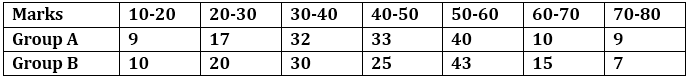Ans. Group A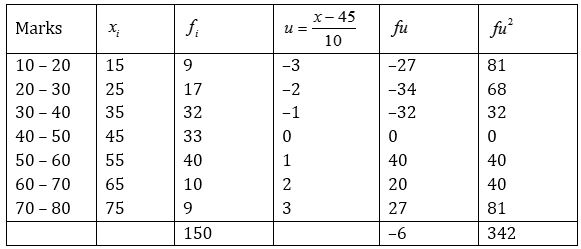Mean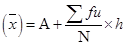=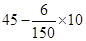= 45 – 0.4 = 44.6

Standard deviation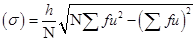=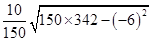=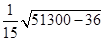=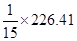= 15.09

Group B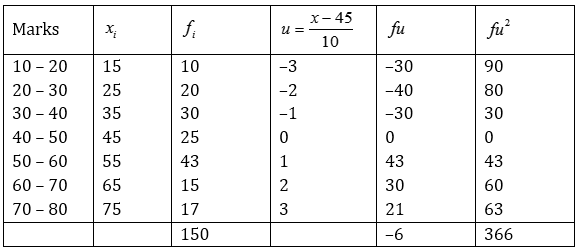Mean== 45 – 0.4 = 44.6

Standard deviation=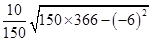=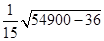=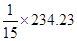= 15.61

2. From the prices of shares X and Y below, find out which is more stable in value: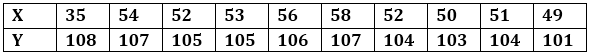Ans.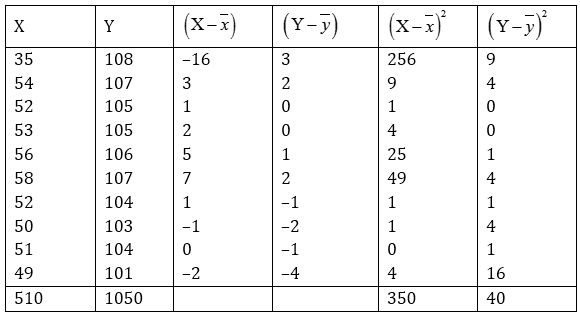Mean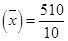= 51and Mean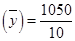105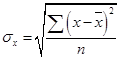=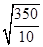= 5.92 and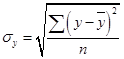=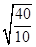= 2

C.V. of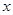=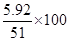= 11.61andC.V. of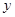=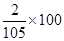= 1.9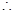C.V. of Y < C.V. of X

Therefore, prices of share Y are more stable.

3. An analysis of monthly wages paid to workers in two firms A and B, belonging to the same industry, gives the following results:

Firm A Firm B

No. of wage earners 586 648

Mean of monthly wages Rs. 5253Rs. 5253

Variance of the distribution of wages 100 121

(i) Which firm A or B larger amount as monthly wages?

(ii) Which firm A or B shows greater variability in individual wages?

Ans. (i) Firm A: Number of wages earners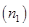= 586

Mean of monthly wages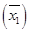= Rs. 5253Total money wages =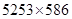= Rs. 3078258

Firm B: Number of wages earners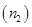= 648

Mean of monthly wages= Rs. 5253Total money wages =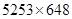= Rs. 3403944

(ii) Since both the firms have same mean of monthly wages, so the firm with greater variance will have more variability in individual wages. Thus firm B will have more variability in individual wages.

4. The following is the record of goals scored by team A in a football session: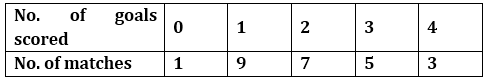For the team B, mean number of goals scored per match was 2 with a standard deviation 1.25 goals. Find which team may be considered more consistent?

Ans. Team A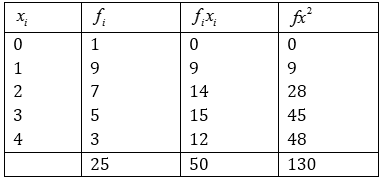Mean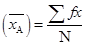=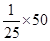= 2

Standard deviation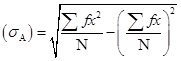=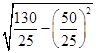=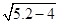=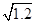= 1.09

C.V. of Team A =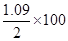= 54.5 and C.V. of Team B =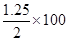= 62.5

Here, C.V. of Team A < C.V. of team B

Therefore, team A is more consistent.

5. The sum and sum of squares corresponding to lengths(in cm) and weight(in gm) of 5- plant products are given below: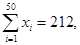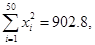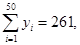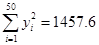Which is more varying, the length or weight?

Ans. Given: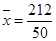= 4.24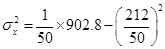= 18.056 – 17.978 = 0.078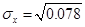= 0.28

Also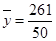= 5.22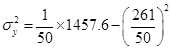= 29.152 – 27.248 = 1.904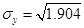= 1.38

C.V. of length =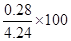= 6.6 and C.V. of weight =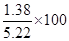Here, C.V of weight > C.V. of length

Therefore, weights have more variability than lengths.### 2 thoughts on “NCERT Solutions class-11 Maths Exercise 15.3”

1. Your way of solving the problem is very easy I like your method from ?

2. Awesome ??? work